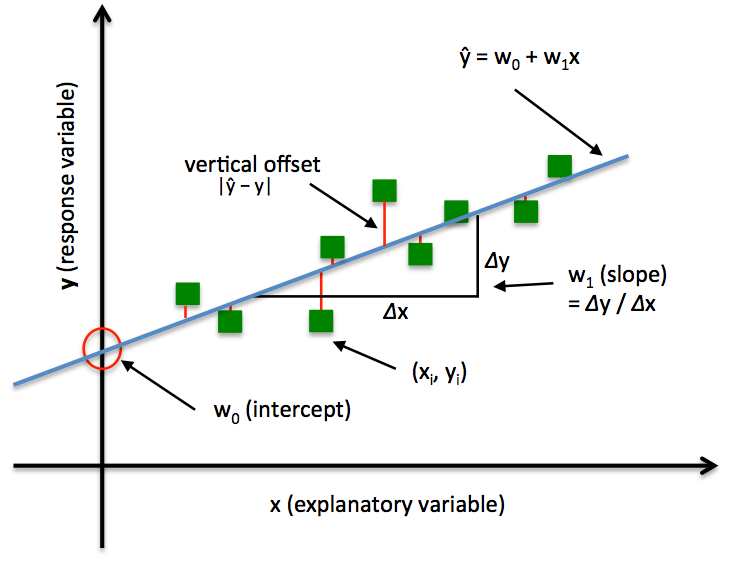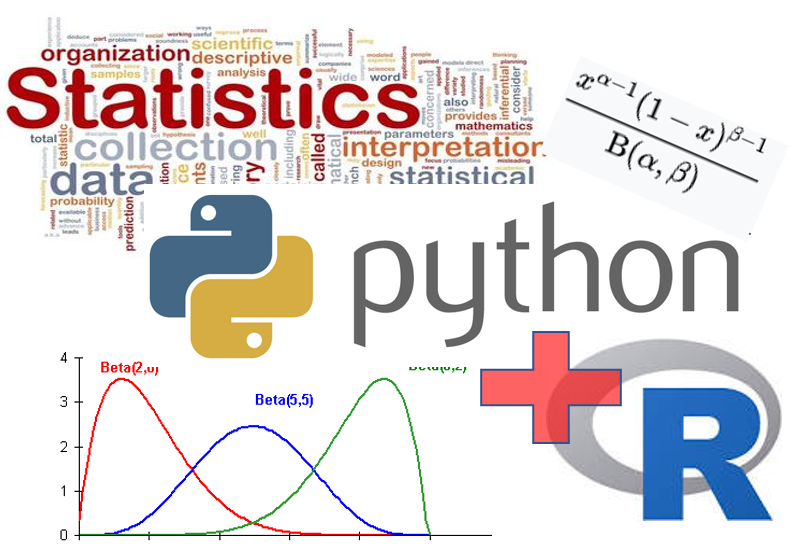Statistics/ Mathematical Computing Notebooks

Jupyter Notebooks on the topics of statistical analysis, mathematics, and numerical/sceintific computing (in Python).

Requirements

• Python 3.6+
• NumPy (\$ pip install numpy)
• Pandas (\$ pip install pandas)
• Scikit-learn (\$ pip install scikit-learn)
• SciPy (\$ pip install scipy)
• Statsmodels (\$ pip install statsmodels)
• MatplotLib (\$ pip install matplotlib)
• Seaborn (\$ pip install seaborn)

Set Algebra basicsPermutations and CombinationsProbability distributions (Discrete)Linear Regression MethodsR-style Statistical functions written using PythonArticles

Check out this article I wrote on Medium: Essential Math for Data Science.

Check out this article I wrote on Medium about "Mathematical programming — a key habit to build up for advancing in data science?"

Check out this article I wrote on Medium about "Bayes’ rule with a simple and practical example"

Check out this article I wrote on Medium about "Statistical modeling with “Pomegranate” — fast and intuitive"

Get A Weekly Email With Trending Projects For These Topics
No Spam. Unsubscribe easily at any time.
Python (1,145,513
Jupyter Notebook (242,614
Machine Learning (31,837
Data Science (9,229
Statistics (4,347
Pandas (3,951
Numpy (3,510
Mathematics (2,714
Analytics (2,270
Matplotlib (2,252
Clustering (1,861
Probability (618
Scipy (564
Numerical Analysis (255
Bayesian Statistics (232
Hypothesis Testing (160
Statsmodels (78
Anova (67
Mathematical Programming (52
Inferential Statistics (31
Normal Distribution (31
Related Projects